### IMO Shortlist 1996 problem A4

Kvaliteta:
Avg: 0,0
Težina:
Avg: 7,0
Let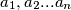$a_{1}, a_{2}...a_{n}$ be non-negative reals, not all zero. Show that that
(a) The polynomial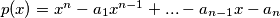$p(x) = x^{n} - a_{1}x^{n - 1} + ... - a_{n - 1}x - a_{n}$ has preceisely 1 positive real root$R$.
(b) let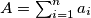$A = \sum_{i = 1}^n a_{i}$ and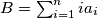$B = \sum_{i = 1}^n ia_{i}$. Show that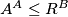$A^{A} \leq R^{B}$.
Izvor: Međunarodna matematička olimpijada, shortlist 1996